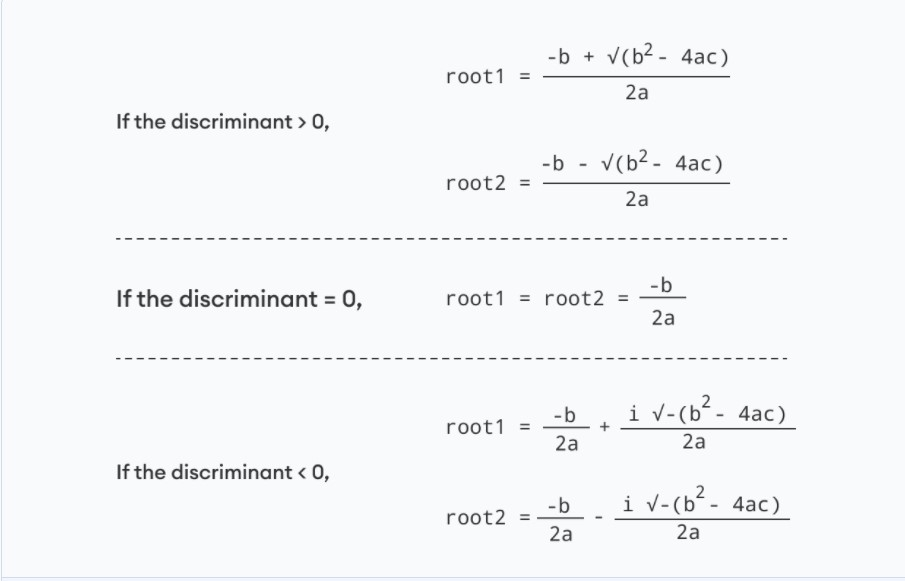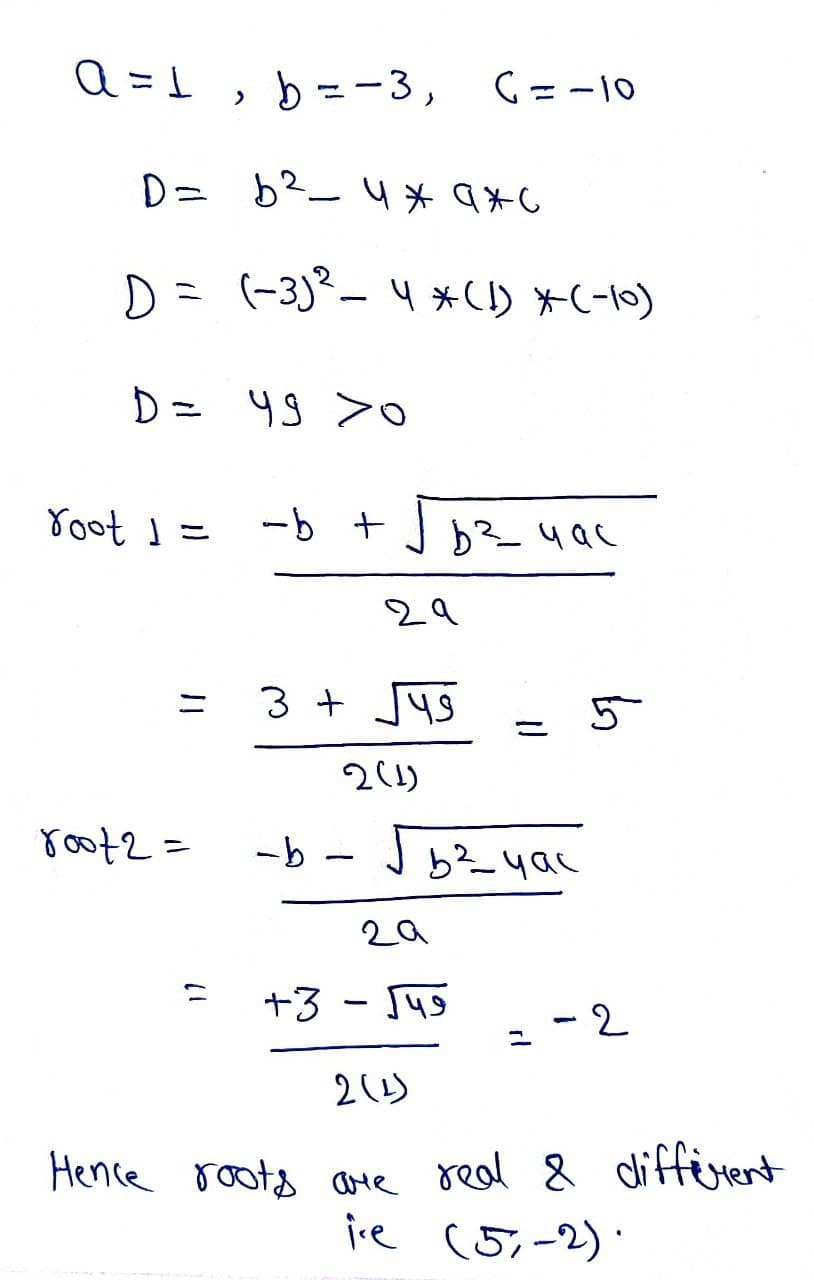# Program to Find Roots of a quadratic equation

Problem Statement: The standard form of a quadratic equation is:

ax2 + bx + c = 0, where a, b and c are real numbers and a != 0

You have given a, b, c of the equation, you have found the roots of the equation.

Examples:

```Example 1:
Input: a = 1, b = -3, c = -10
Output: Roots are real and different, i.e(5 , -2).

Example2:

Input: a = 1, b = 1, c = 1
Output: Roots are complex, i.e-(-0.5+i1.732 , -0.5-i1.732).
```

### Solution

Disclaimer: Don’t jump directly to the solution, try it out yourself first.

Solution: Using Discriminant and formula

Intuition: For finding out the roots of the equation we have to find the discriminant of the equation, which tells the nature of the roots.

Then use the formula of finding the roots.

Approach:

• Find discriminant of the equation.
• Discriminant(D) = b^2 – 4a*c
• If the discriminant is greater than 0, the roots are real and different.
• If the discriminant is equal to 0, the roots are real and equal.
• If the discriminant is less than 0, the roots are complex and different.

Then roots are:Dry run:Code:

## C++ Code

``````#include <bits/stdc++.h>
using namespace std;

void Roots(int a, int b, int c)
{
int d = b * b - 4 * a * c;
double sqrt_val = sqrt(abs(d));

if (d > 0) {
cout << "Roots are real and different \n";
double root1 = (double)(-b + sqrt_val) / (2 * a);
double root2 = (double)(-b - sqrt_val) / (2 * a);
cout << root1 <<"\n"<< root2;
}
else if (d == 0) {
cout << "Roots are real and same \n";
double root1 = -(double)b / (2 * a);
double root2 = -(double)b / (2 * a);
cout << root1 <<"\n" <<root2;
}
else // d < 0
{
cout << "Roots are complex \n";
cout << -(double)b / (2 * a) << " + i" << sqrt_val
<< "\n"
<< -(double)b / (2 * a) << " - i" << sqrt_val;
}
}

int main()
{
int a = 1, b = -3, c = -10;
Roots(a, b, c);

return 0;
}
``````

Output:

Roots are real and different
5
-2

Time Complexity: O(N)

Space Complexity: O(1)

## Java Code

``````import java.util.*;

public class tuf {
static void Roots(int a, int b, int c)
{
if (a == 0) {
System.out.println("Invalid");
return;
}

int d = b * b - 4 * a * c;
double sqrt_val = Math.sqrt(Math.abs(d));

if (d > 0) {
System.out.println("Roots are real and different ");

double root1 = (double)(-b + sqrt_val) / (2 * a);
double root2 = (double)(-b - sqrt_val) / (2 * a);
System.out.println(root1 + "\n"+root2);
}
else if (d == 0) {
System.out.println("Roots are real and same ");
double root1 = -(double)b / (2 * a);
double root2 = -(double)b / (2 * a);
System.out.println(root1 + "\n"+root2);
}
else // d < 0
{
System.out.println("Roots are complex ");

System.out.println(-(double)b / (2 * a) + " + i"+ sqrt_val + "\n"+
-(double)b / (2 * a) + " - i" + sqrt_val);
}
}
public static void main(String args[])
{
int a = 1, b = -3, c = -10;

Roots(a, b, c);
}
}
``````

Output:

Roots are real and different
5.0
-2.0

Time Complexity: O(N)

Space Complexity: O(1)

Special thanks to Prashant Sahu for contributing to this article on takeUforward. If you also wish to share your knowledge with the takeUforward fam, please check out this article